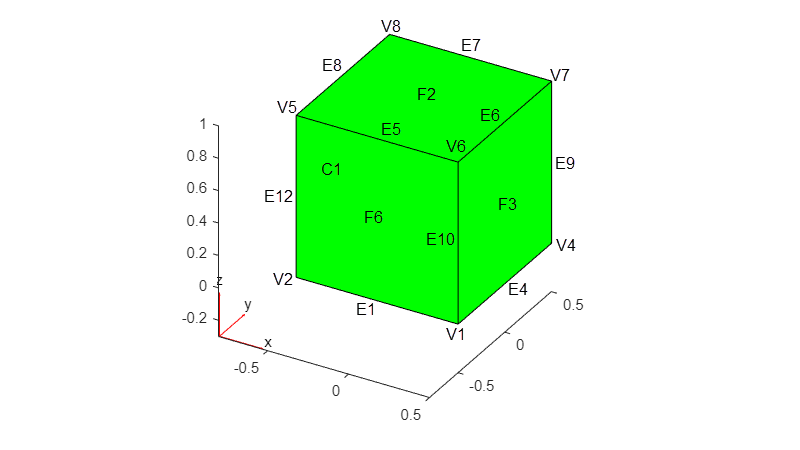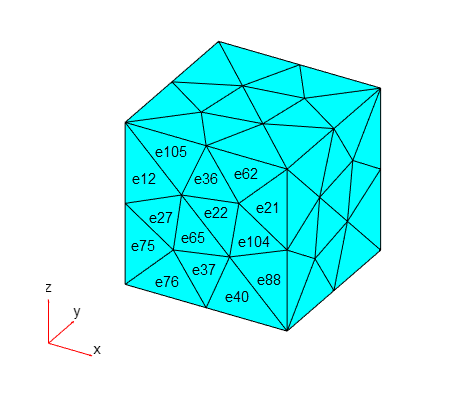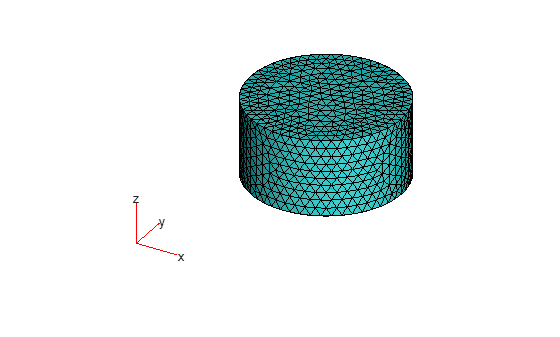# Geometry and Mesh Components

This example shows how the toolbox represents geometries and meshes, the components of geometries and meshes, and the relationships between them within a model object.

### Geometry

The toolbox supports 2-D and 3-D geometries. Each geometry in the toolbox consists of these components, also called geometric regions: vertices, edges, faces, and cells (for a 3-D geometry). Each geometric region has its own label that follows these conventions:

• Vertex labels — Letter `V` and positive integers starting from 1

• Edge labels — Letter `E` and positive integers starting from 1

• Face labels — Letter `F` and positive integers starting from 1

• Cell labels — Letter `C` and positive integers starting from 1

For example, the toolbox represents a unit cube geometry with these geometric regions and labels:

• Eight vertices labeled from `V1` to `V8`

• Twelve edges labeled from `E1` to `E12`

• Six faces labeled from `F1` to `F6`.

• One cell labeled `C1`

Numbering of geometric regions can differ in different releases. Always check that you are assigning parameters of a problem to the intended geometric regions by plotting the geometry and visually inspecting its regions and their labels.To set up a PDE problem, the toolbox combines a geometry, mesh, PDE coefficients, boundary and initial conditions, and other parameters into a model object. A geometry can exist outside of a model. For example, create a unit sphere geometry.

`gm1 = multisphere(1)`
```gm1 = DiscreteGeometry with properties: NumCells: 1 NumFaces: 1 NumEdges: 0 NumVertices: 0 Vertices: [] ```

You can also import a geometry.

`gm2 = importGeometry("Block.stl")`
```gm2 = DiscreteGeometry with properties: NumCells: 1 NumFaces: 6 NumEdges: 12 NumVertices: 8 Vertices: [8x3 double] ```

When a geometry exists within a model, the toolbox stores it in the `Geometry` property of the model object. For example, create a model and assign the unit sphere geometry `gm1` to its `Geometry` property.

```model1 = createpde; model1.Geometry = gm1```
```model1 = PDEModel with properties: PDESystemSize: 1 IsTimeDependent: 0 Geometry: [1x1 DiscreteGeometry] EquationCoefficients: [] BoundaryConditions: [] InitialConditions: [] Mesh: [] SolverOptions: [1x1 pde.PDESolverOptions] ```

You also can import a geometry and assign it to the `Geometry` property of a model in one step by using `importGeometry`.

```model2 = createpde; importGeometry(model2,"Block.stl")```
```ans = DiscreteGeometry with properties: NumCells: 1 NumFaces: 6 NumEdges: 12 NumVertices: 8 Vertices: [8x3 double] ```

### Mesh

A mesh approximates a geometry and consists of elements and nodes. The toolbox uses meshes with triangular elements for 2-D geometries and meshes with tetrahedral elements for 3-D geometries.

Triangular elements in 2-D meshes are specified by three nodes for linear elements or six nodes for quadratic elements. A triangle representing a linear element has nodes at the corners. A triangle representing a quadratic element has nodes at its corners and edge centers.

Tetrahedral elements in 3-D meshes are specified by four nodes for linear elements or 10 nodes for quadratic elements. A tetrahedron representing a linear element has nodes at the corners. A tetrahedron representing a quadratic element has nodes at its corners and edge centers.

Each mesh component has its own label that follows these conventions:

• Mesh element labels — Letter `e` and positive integers starting from 1

• Mesh node labels — Letter `n` and positive integers starting from 1

The mesh generator can return slightly different meshes in different releases. For example, the number of elements in the mesh can change. Write code that does not rely on explicitly specified node and element IDs or node and element counts.### Relationship Between Geometry and Mesh

Geometric regions do not describe a mesh or its elements. A geometry can exist outside of a model, while a mesh is always a property of the model.

```gm = multicuboid(1,1,1); model = createpde; model.Geometry = gm; generateMesh(model,"Hmin",0.5); model```
```model = PDEModel with properties: PDESystemSize: 1 IsTimeDependent: 0 Geometry: [1x1 DiscreteGeometry] EquationCoefficients: [] BoundaryConditions: [] InitialConditions: [] Mesh: [1x1 FEMesh] SolverOptions: [1x1 pde.PDESolverOptions] ```

A geometry, even when it is a property of a model, is stored separately from a mesh. The toolbox does not automatically regenerate the mesh when you modify a geometry.

```new_gm = multicylinder(1,1); model.Geometry = new_gm; pdegplot(model,"FaceAlpha",0.3) hold on pdemesh(model)```You must explicitly update the mesh to correspond to the current cylinder geometry.

```generateMesh(model); pdegplot(model,"FaceAlpha",0.3) hold on pdemesh(model)```### Geometry and Mesh Queries

The toolbox enables you to find mesh elements and nodes by their geometric location or proximity to a particular point or node. For example, you can find all elements that belong to a particular face or cell. You also can find all nodes that belong to a particular vertex, edge, face, or cell. For details, see `findElements` and `findNodes`.

The toolbox also enables you to find edges and faces by their proximity to a particular point or to find only those attached to a particular geometric region:

### Parameters of a Model on Geometric Regions

The toolbox lets you specify parameters of each problem, such as boundary and initial conditions (including boundary constraints and boundary loads) and PDE coefficients (including properties of materials, internal heat sources, body loads, and electromagnetic sources) on geometric regions. For example, you can apply boundary conditions on the top and bottom faces of this cylinder. First, create the cylinder geometry.

```gm = multicylinder(5,10); pdegplot(gm,"FaceLabels","on","FaceAlpha",0.5);```Then, create a model, add the geometry to the model, and apply the Dirichlet boundary conditions on the top and bottom faces of the cylinder.

```model = createpde; model.Geometry = gm; applyBoundaryCondition(model,"dirichlet","Face",1:2,"u",0)```
```ans = BoundaryCondition with properties: BCType: 'dirichlet' RegionType: 'Face' RegionID: [1 2] r: [] h: [] g: [] q: [] u: 0 EquationIndex: [] Vectorized: 'off' ```

### Solvers Use Meshes

PDE solvers do not work with geometries directly. They work with the corresponding meshes instead. For example, if you generate a coarse mesh, the PDE solver uses the discretized cylinder.

```generateMesh(model,"Hmin",4); figure pdemesh(model)```When you solve a problem, the toolbox internally finds all mesh nodes and elements that belong to these geometric regions and applies the specified parameters to those nodes and elements. The discretized top and bottom of the cylinder look like polygons rather than circles.

```figure pdemesh(model) view([34 90])```When you refine a mesh for your problem, the toolbox automatically recalculates which nodes and elements belong to particular geometric regions and applies the specified parameters to the new nodes and elements.

```generateMesh(model); figure pdemesh(model) view([34 90])```Although the solvers apply specified parameters to mesh elements and nodes, you cannot explicitly specify these parameters directly on mesh components. All parameters must be specified on geometric regions. This approach prevents unintended assignments that can happen, for example, when you refine a mesh.